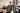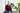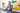20.10.2021 |

# Abstract Factory

https://codesandbox.io/s/zen-voice-u21en

# Square game

Imagine we want to build a game where you can create squares with your mouse. In every level the squares should have a different look and behaviour. The core game loop should stay the same. What's the best way to accomplish this?

# Abstract Factory

You guessed correctly, the abstract factory pattern!

``````interface SquareFactory {
makeSquare(x: number, y: number): Square;
}

interface Square {
x: number;
y: number;

update(): void;

draw(canvas: HTMLCanvasElement): void;
}``````

Our game loop can use this factory to create a square when the user clicks the screen.

``````
class MouseSquarePainter {
squares: Square[] = [];

constructor(private canvas: HTMLCanvasElement, private sf: SquareFactory) {
if (e.buttons === 1) {
e.preventDefault();
}
});

this.update();
}

const sq = this.sf.makeSquare(x, y);
sq.draw(this.canvas);
this.squares.push(sq);
}

update() {
[...]

this.squares.forEach((s) => {
s.draw(this.canvas);
});

requestAnimationFrame(() => this.update());
}
}``````

# Implementation

To create a level we now have to implement our SquareFactory and Square interfaces.

``````class BigBlueSquare implements Square {
speedX = Math.random() - 0.5;
speedY = Math.random() - 0.5;

constructor(public x: number, public y: number) {}

update() {
this.speedX *= 1.01;
this.x += this.speedX;
this.speedY *= 1.01;
this.y += this.speedY;
}

draw(canvas: HTMLCanvasElement) {
const ctx = canvas.getContext("2d")!;
ctx.strokeStyle = "#FF0000";
ctx.fillStyle = "#0000FF55";
ctx.beginPath();
ctx.rect(this.x - 25, this.y - 25, 50, 50);
ctx.stroke();
ctx.fill();
}
}

class BigBlueSquareFactory implements SquareFactory {
makeSquare(x: number, y: number) {
return new BigBlueSquare(x, y);
}
}``````

And pass an instance of our Factory to the game.

``const rsp2 = new MouseSquarePainter(canvas2, new BigBlueSquareFactory());``

View the full code and play the "game" here: https://codesandbox.io/s/zen-voice-u21en?file=/src/index.ts